# QlikView Layout & Visualizations

Discussion Board for collaboration on QlikView Layout & Visualizations.

Highlighted
New Contributor II

## Divide measure before date X

Hello all,

I really need some help, I have the following chart: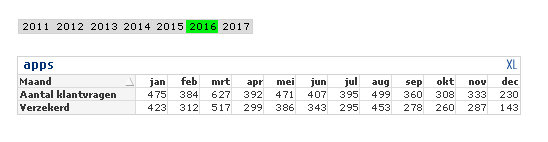formulas are:

Aantal klantvragen: count({<[Klant label]={'XX'},[Month]=>}AppID)

Verzekerd: count({<[Klant label]={'XX'},[IsVerzekerde]={1},[Month]=>}AppID)

What I want and can't get working. I would like to divide al numbers before Date 25-11-2016 by 2. And round up.

numbers would be like:

Aantal klantvragen:

jan 238

feb 192

mrt 314

dec 230

I have a Date field in format 'DD-MM-YYYY'.

I hope someone can help me.

Thanks!

Regards Stefan

1 Solution

Accepted SolutionsMVP

## Re: Divide measure before date X

Try may be using Aggr() function around this

Sum(Aggr(If(MakeDate(2016, 11, 25) < Date, Count({<[Klant label]={'XX'},[Month]=>}AppID)/2, Count({<[Klant label]={'XX'},[Month]=>}AppID)), Date, Maand))

But since you are doing this by date and not month, you will have more than half of the month of december where the result is divided by 2. Is that what you want?

10 RepliesPartner

## Re: Divide measure before date X

Hi Stefan,

Have you tried;

if(Date < '25-11-2016', count({<[Klant label]={'XX'},[Month]=>}AppID) /2, count({<[Klant label]={'XX'},[Month]=>}AppID))MVP

## Re: Divide measure before date X

May be like this?

If(Month(MakeDate(2016, 11, 25)) < Maand, Count({<[Klant label]={'XX'},[Month]=>}AppID)/2, Count({<[Klant label]={'XX'},[Month]=>}AppID))

New Contributor II

## Re: Divide measure before date X

Thanks,

I've changed this to:

If(MakeDate(2016, 11, 25) < Date, Count({<[Klant label]={'XX'},[Month]=>}AppID)/2, Count({<[Klant label]={'XX'},[Month]=>}AppID))

Now it works when I select one date, when I select more dates I get the old numbers.

Any ideas?

Thanks, StefanMVP

## Re: Divide measure before date X

Still trying to understand how this is being used.... Can you share images of how it looks when you select a single date and how it looks when you select multiple dates? May be a sample might help speed up things

New Contributor II

## Re: Divide measure before date X

Hi Sunny,

Sample is not easy because of non disclosure agreement, scrambling screws up my set analysis.

I will try it with more images:

1) No selection on date gives bad result: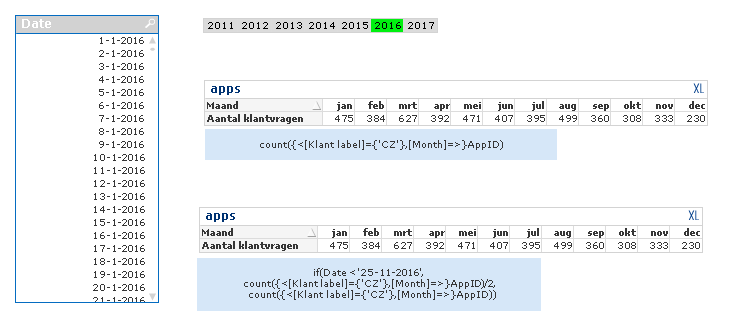2) selection on date 1-1-2016 gives good result (15/2 = 7.5) :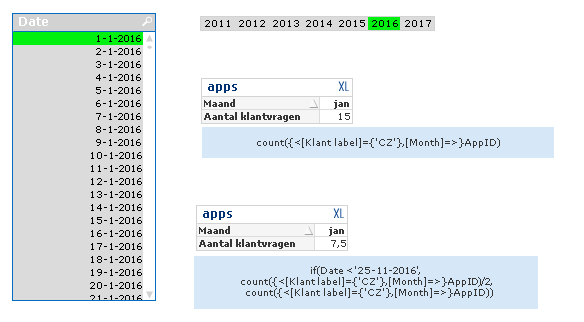3) selection on 2-1-2016 gives good result (16/2 = 8):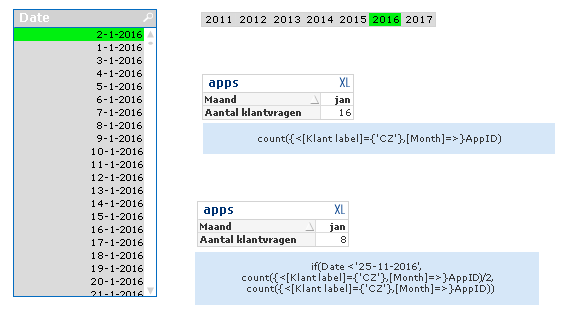4) selection on 1-1-2016 and 2-1-2016 gives bad result: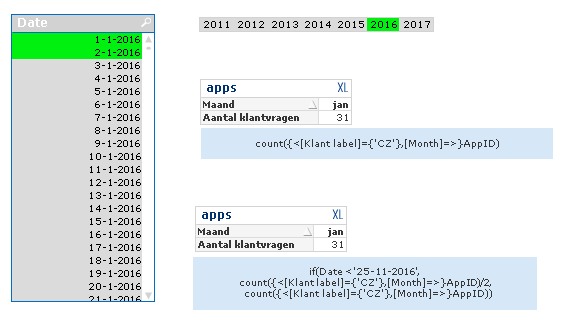So it only works when there is one date selected. The goal is that everything up to 25-11-2016 is divided by two (even without selections).

Regards,

StefanMVP

## Re: Divide measure before date X

Try may be using Aggr() function around this

Sum(Aggr(If(MakeDate(2016, 11, 25) < Date, Count({<[Klant label]={'XX'},[Month]=>}AppID)/2, Count({<[Klant label]={'XX'},[Month]=>}AppID)), Date, Maand))

But since you are doing this by date and not month, you will have more than half of the month of december where the result is divided by 2. Is that what you want?

New Contributor II

## Re: Divide measure before date X

You mean more than half of the month november?

New Contributor II

## Re: Divide measure before date X

This is it, great! Thanks!MVP

## Re: Divide measure before date X

Right, that's what I meant